# Missing DI

### D.1-5) Study the following table carefully and answer the following questions given below.

Given table shows that the speed of the train, car, man and bike. you have to calculate the missing data by using the given data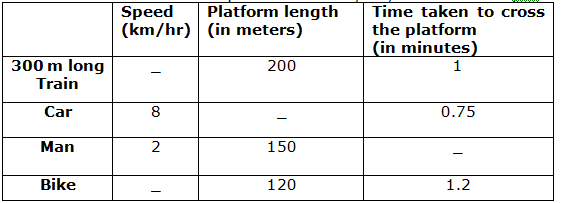Q.1) Two friends A and B starts at 8.00a.m and travel by train from point X to point Y. After travelling a certain distance, train breaks down, say at point Z. Both of them proceed further to point Y.A travels by car. B starts walking and after covering  of the residual distance is picked up by C on bike. A reaches point Y 9 hours before B and C reach there. If the Distance between point X and point Y is 120 km. find the time of breakdown of the train

a) 9.25.a.m

b) 10.42.a.m

c) 9.36.a.m

d) 10.a.m

e) 11.a.m

(c)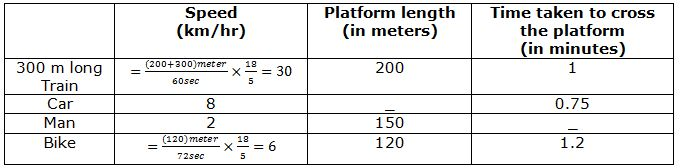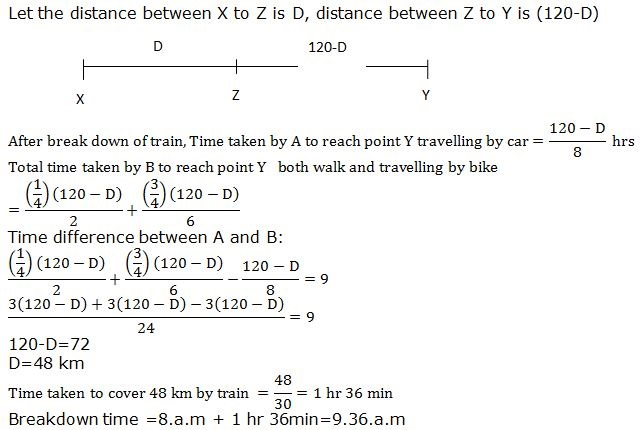Q.2) A man usually goes to his office by car. One day as the car had breakdown, he went to office by bike which took 2hrs longer than had he travelled by car. Next day he travels by bike in the alternate path, he takes 4 hrs less of time than the earlier day. Find the difference between the distance travelled in the usual route and alternate route?

a) 48 km

b) 24 km

c) 32 km

d) 30 km

e) None of these

(c)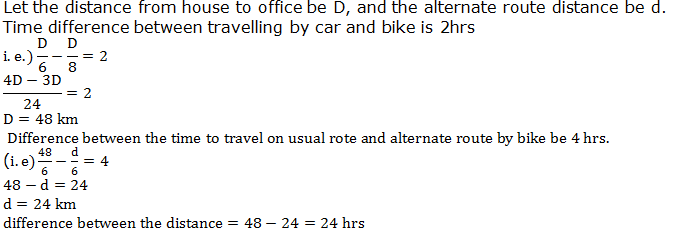Q.3) Two cyclist starts from the same place and ride in the same direction. P starts at 12.00 noon and Q starts at 1.30 p.m. Speed of cyclist P is equal to the speed of the car and speed of cyclist Q is 2km/hr more than that of P. How far will P have travelled before he is overtaken by Q?

a) 70 km

b) 60 km

c) 45 km

d) 55 km

e) 30 km

(b)Speed of cyclist P = 8 km/hr

Speed of cyclist Q =10 km/hr

If P rides for x hours before he is overtaken, then B rides for (x-1.5) hrs.

8x=10(x-1.5)

X=7.5 hrs

Distance=7.5×8=60 km

Q.4) A man covers a certain distance on car. Had he moved 4 km/hr faster, he would have taken 20 min less. If he had moved 2 km/hr slower he would have taken 20min more. What is the distance?

a) 10 km

b) 12 km

c) 8 km

d) 6 km

e) None of these

(c)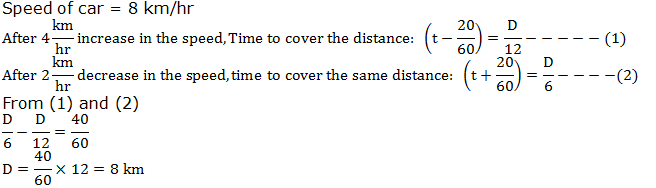Q.5) A man rides one fourth of the distance from A to B by walk and the remaining distance by bike. Total time taken is 30 hrs more than the time taken by the car to travel A to B. Find the distance between A and B?

a) 240 km

b) 120 km

c) 200 km

d) 160 km

e) 180 km

(a)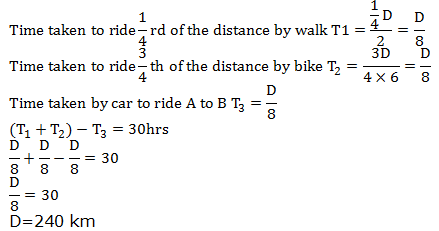### D.6-10) Study the following information carefully and answer the question given below.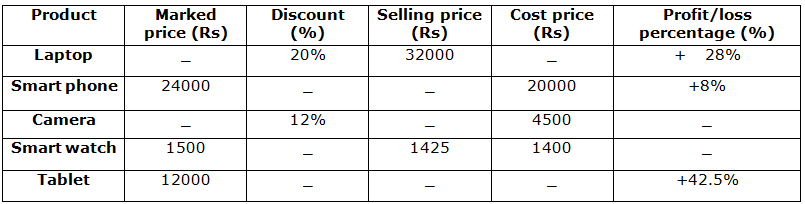Q.6) Selling price of smart phone is what percent of cost price of Tablet, if the discount rate of tablet is half of the discount rate of smart phone?

a) 238%

b) 280%

c) 242%

d) 270%

e) 286%

(d)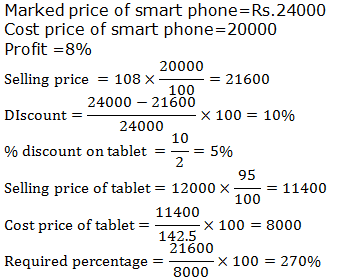Q.7)  If there has been a loss of 42.5% on Tablet instead of 42.5% profit. Then the new selling price is how much less than the original S.P.? (Approximate)

(Take the discount rate of table from the above question)

a) 65%

b) 60%

c) 55%

d) 53%

e) None of these

(b)Q.8) If the ratio between marked price of laptop and camera is 8:1, then the profit/loss percentage on smart watch is approximately what percent more/less than profit/loss percentage on camera?

a) 20% more

b) 18% more

c) 19% less

d) 20% less

e) 19% more

(c)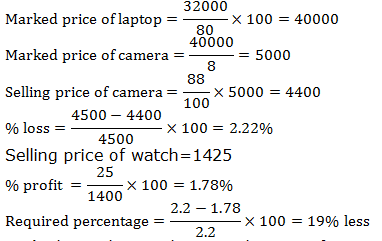Q.9) What is the ratio between discount of smart watch and discount of tablet if the selling price of tablet is 9975 more than the selling price of smart watch?

a) 5 : 3

b) 1:1

c) 3 : 5

d) 2 : 5

e) 1:2

(b)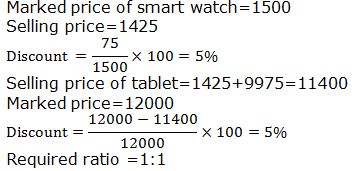Q.10) What is the difference between the total cost price and total selling price of all 5 products (In Rs)?

(Take the discount rate of table found in question number 27)

a) 11935

b) 12050

c) 11950

d) 11800

e) 11925

(e)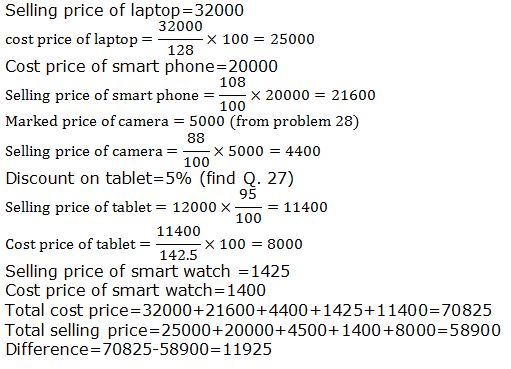Selling price of laptop=32000

Cost price of smart phone=20000

Discount on tablet=5% (find Q. 27)

Selling price of smart watch =1425

Cost price of smart watch=1400

Total cost price=32000+21600+4400+1425+11400=70825

Total selling price=25000+20000+4500+1400+8000=58900

Difference=70825-58900=11925